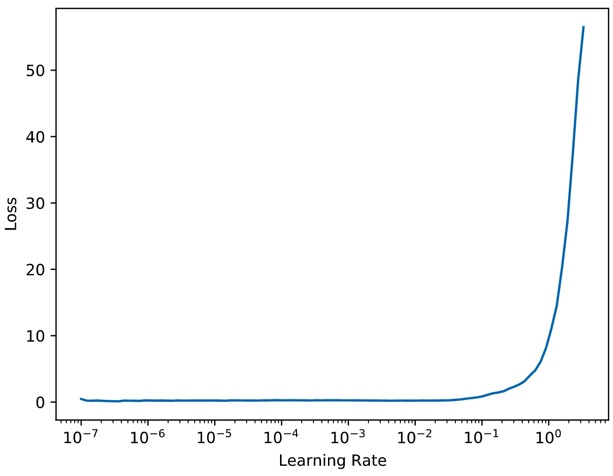# Lr_find() not completing

I’m attempting to do the dog breeds challenge, and after I call the following code, I call learn.lr_find().

!rm -rf {PATH}tmp
data = ImageClassifierData.from_paths(PATH, tfms=tfms_from_model(arch, sz))
learn = ConvLearner.pretrained(arch, data, precompute=True)

When I call learn.lr_find(), it starts to calculate, but stops when it gets to about 87%. When I try to print it out, it says that it returned None. Am I calling it properly, or is there some other possible reason?

It doesn’t return anything. Have a look at how we use it in lesson1.ipynb. It doesn’t finish, because it stops once the loss gets much worse.

4 Likes

Thanks professor! It looks like I forgot to open one of the hidden tabs in the lesson1 jupyter page, and I missed the description on how it was used. I forgot about how you can plot the learning rate and find the best one based on the point where it’s still improving but really close to where it needs to be.

Just for anybody else that sees this and has questions, after doing the `learn.lr_find()` command, you have to do `learn.sched.plot()` to plot what that looks like and determine the learning rate you should use. And just a reminder, the learning rate you choose should be where the curve is still sloping down and not at the very bottom of the graph. Also something I screwed up on to start, these plots are on a log scale, so keep that in mind when determining the learning rate to use.

1 Like

Hi,

Sorry to dig up an old post, but this behaviour still happens in V2.

I was running a transfer learning model on a small image classification dataset, I reloaded the model after 10k epochs, and went through my pipeline where ran the lr_find function first, and use the suggestions to guide the one cycle policy. What I have found is that if the stop_div=True, then occasionally I had a case where the learning rate diverged after one step, so the LRfinder callback called it quits, the record logged only one lr and loss value, and therefore didn’t return a learning rate suggestion.

If I set stop_div=False, it worked fine… Here is what the learning rate finder graph looks like (if I run it with stop_div = False):Note, This is actually using a model that has already been trained for 10k epochs on a small dataset, so maybe I shouldn’t be running the learning rate finder.

However,

Would it make sense to flag an error in the lr find if it exits after one iteration due to divergence and therefore doesn’t return suggested learning rates? Should I submit this as a bug/ feature request?

Cheers,

Jon